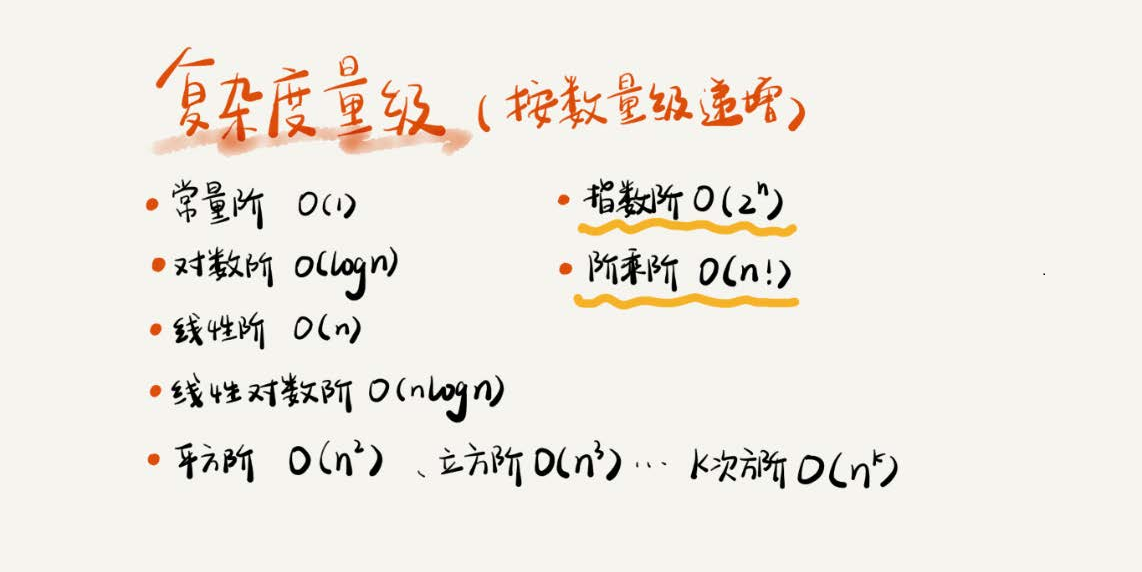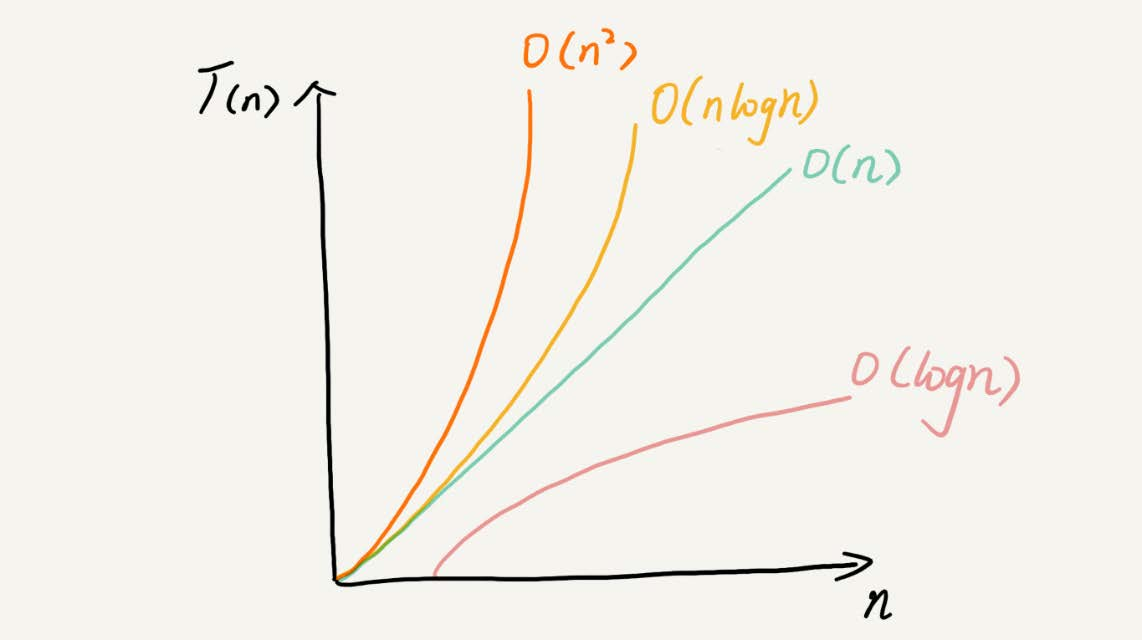# 复杂度分析（笔记）

03复杂度分析（上）：如何分析、统计算法的执行效率和资源消耗？

## 1.为什么需要复杂度分析？

1.测试结果非常依赖测试环境

• 测试环境中硬件的不同会对测试结果产生很大的影响。比如，分别用i9处理器和i3处理器运行。还有，a代码和b代码在不同机器上的运行速度可能会有截然相反的结果。

2.测试结果受数据规模大小的影响

• 举个例子，同一个排序算法，待排序数据的有序度不一样，排序的执行时间会有很大差别。极端情况下，如果数据已经近乎有序，排序算法几乎不需要做任何操作，执行时间就会非常短。如果测试数据规模太小，测试结果可能无法真实地反映算法的性能。比如，对小规模的数据排序，插入排序可能比快速排序更快。

## 2.大O复杂度表示法

int cal(int n){
int sum = 0;
int i = 1;
for(; i <= n; ++i){
sum = sum + i;
}
return sum;
}


(2n+2)*unit_time。代码的执行时间T(n)与每行代码的执行次数成正比。

int cal(int n){
int sum = 0;
int i = 1;
int j = 1;
for(; i <= n; ++i){
j = 1;
for(; j <= n; ++j){
sum = sum + i * j;
}
}
return sum;
}


## 3.时间复杂度分析

1.只关注循环执行次数最多的一次代码。

• 刚才说了，大O复杂度表示方法只表示一种变化趋势。我们通常会忽略掉公式中的常量、低阶、系数，只需要记录一个最大阶的量级就可以了。所以，我们在分析一个算法、一段代码的时间复杂度的时候，只关注循环执行次数最多的那一段代码就可以了。这段核心代码执行次数的n的量级，就是整段要分析代码的时间复杂度。为了便于理解，拿例子来说明。
int cal(int n){
int sum = 0;
int i = 1;
for(; i <= n; ++i){
sum = sum + i;
}
return sum;
}


2.加法法则：总复杂度等于量级最大的那段代码的复杂度。

• 这里还有一段代码。先试着分析一下，然后再往下看分析思路。
int cal(int n) {
int sum_1 = 0;
int p = 1;
for (; p < 100; ++p) {
sum_1 = sum_1 + p;
}

int sum_2 = 0;
int q = 1;
for (; q < n; ++q) {
sum_2 = sum_2 + q;
}

int sum_3 = 0;
int i = 1;
int j = 1;
for (; i <= n; ++i) {
j = 1;
for (; j <= n; ++j) {
sum_3 = sum_3 + i * j;
}
}

return sum_1 + sum_2 + sum_3;
}


3.乘法法则：嵌套代码的复杂度等于嵌套内外代码复杂度的乘积

• 刚讲了一个复杂度分析中的加法法则，这儿还有一个乘法法则。类比一下，你应该能“猜到”公式。如果T1(n)=O(f(n))，T2(n)=O(g(n))；那么T(n)=T1(n)*T2(n)=O(f(n))*O(g(n))=O(f(n)*g(n))。

int cal(int n) {
int ret = 0;
int i = 1;
for (; i < n; ++i) {
ret = ret + f(i);
}
}

int f(int n) {
int sum = 0;
int i = 1;
for (; i < n; ++i) {
sum = sum + i;
}
return sum;
}


## 4.几种常见时间复杂度分析1.O(1)

• 必须明确一个概念，O(1)只是常量级时间复杂度的一种表示方法，并不是指只执行了一行代码。这段代码即便有3行，它的时间复杂度也是O(1），而不是O(3)。
int i = 8;
int j = 6;
int sum = i + j;


2.O(logn)、O(nlogn)

• 对数阶时间复杂度非常常见，也是最难分析的一种时间复杂度，举例说明。
i=1;
while (i <= n) {
i = i * 2;


i=1;
while (i <= n) {
i = i * 3;
}


3.O(m+n)、O(m*n)

• 再来讲一种跟前面都不一样的时间复杂度，代码的复杂度由两个数据的规模来决定。老规矩，先看代码！
int cal(int m, int n) {
int sum_1 = 0;
int i = 1;
for (; i < m; ++i) {
sum_1 = sum_1 + i;
}

int sum_2 = 0;
int j = 1;
for (; j < n; ++j) {
sum_2 = sum_2 + j;
}

return sum_1 + sum_2;
}


## 5.空间复杂度分析

void print(int n) {
int i = 0;
int[] a = new int[n];
for (i; i <n; ++i) {
a[i] = i * i;
}

for (i = n-1; i >= 0; --i) {
print out a[i]
}
}


## 6.内容小结复杂度分析并不难，关键在于多练。每次看到代码的时候，简单的一眼就能看出其复杂度，难的稍微分析一下就能得出答案。（多思考多练习）

08-31114208-2032
08-1032
08-2041
04-191199
09-28535
08-2157
10-2938
04-0145
11-17462